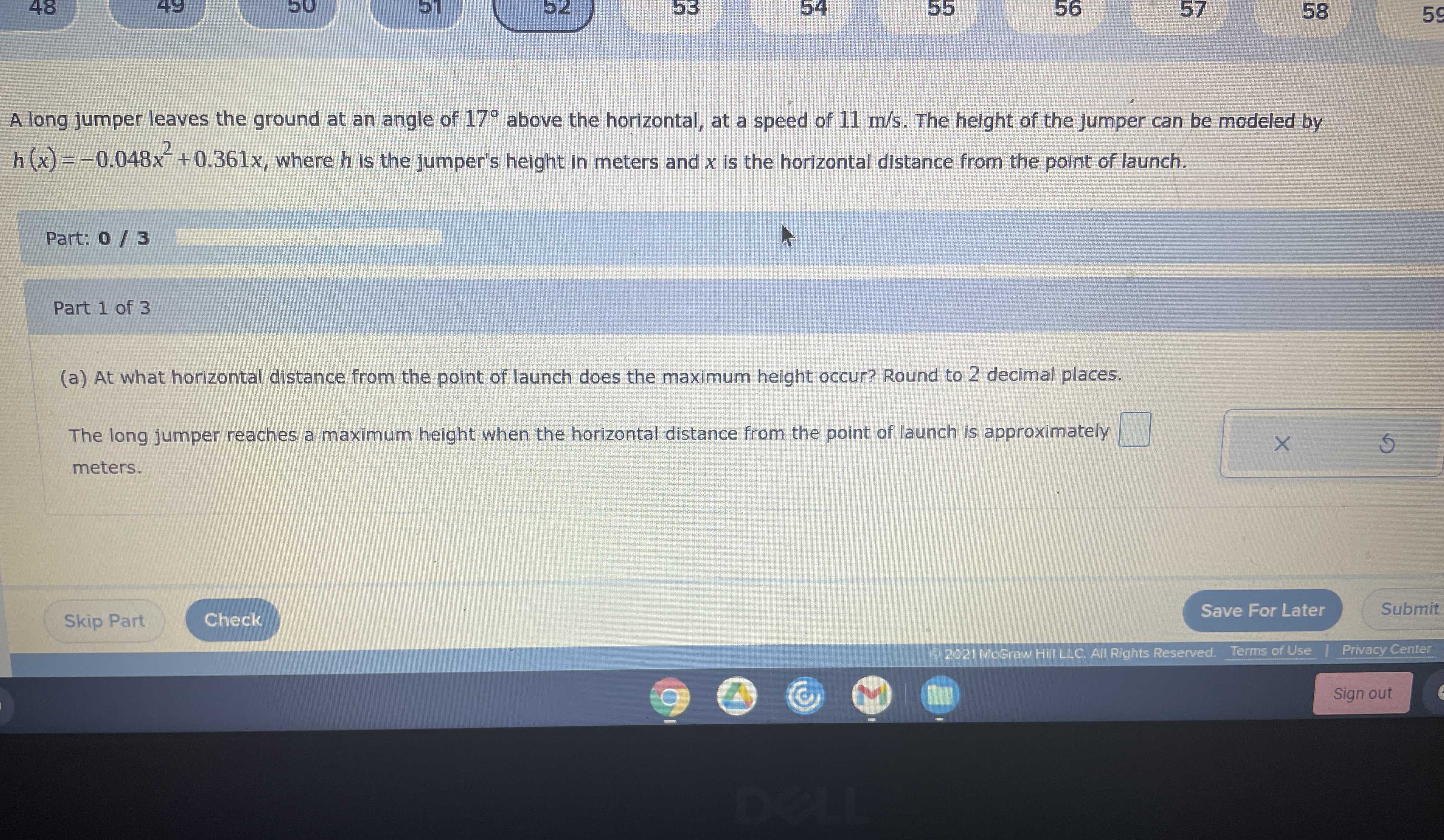### ¿Todavía tienes preguntas de matemáticas?

Pregunte a nuestros tutores expertos
Algebra
PreguntaA long jumper leaves the ground at an angle of $$17 ^ { \circ }$$ above the horizontal, at a speed of $$11 m / s$$ . The height of the jumper can be modeled by $$h ( x ) = - 0.048 x ^ { 2 } + 0.361 x$$ , where $$h$$ is the jumper's height in meters and $$x$$ is the horizontal distance from the point of launch.

(a) At what horizontal distance from the point of launch does the maximum height occur? Round to $$2$$ decimal places. The long jumper reaches a maximum height when the horizontal distance from the point of launch is approximately $$\square$$ meters.